Questions from Inside the chapter

Class 10
Chapter 12 Class 10 - Electricity

## An electric lamp of 100 Ω, a toaster of resistance 50 Ω, and a water filter of resistance 500 Ω are connected in parallel to a 220 V source. What is the resistance of an electric iron connected to the same source that takes as much current as all three appliances, and what is the current through it?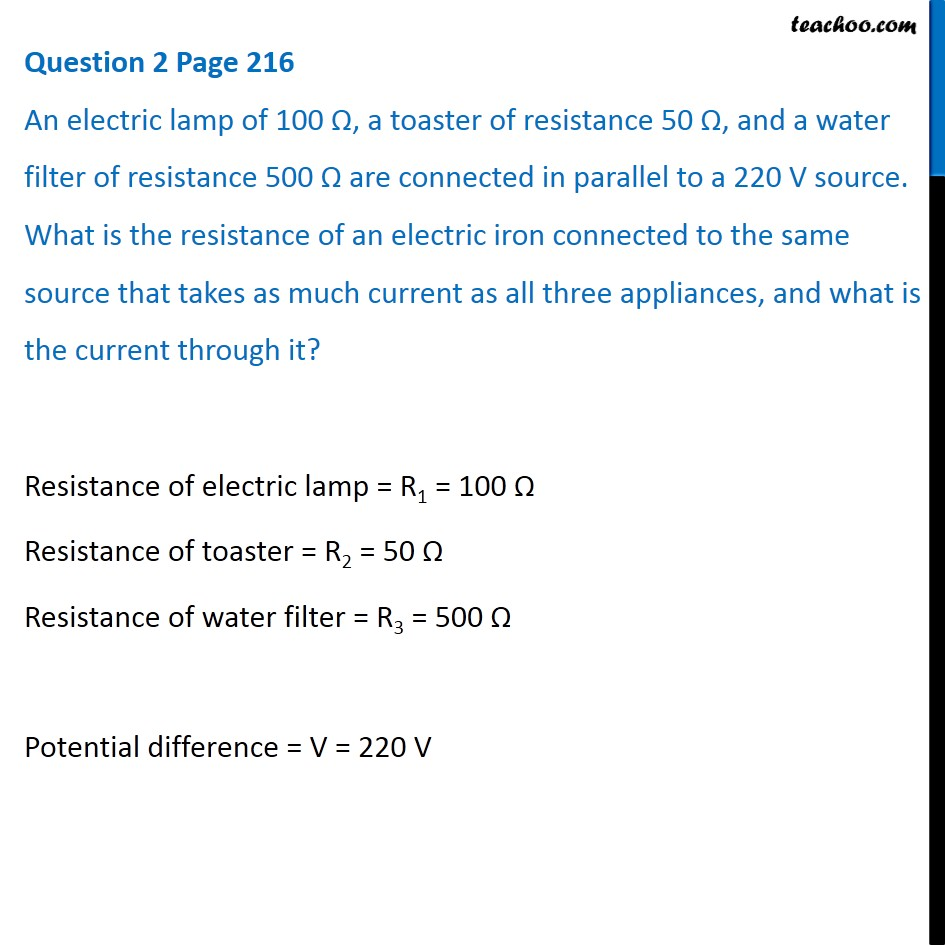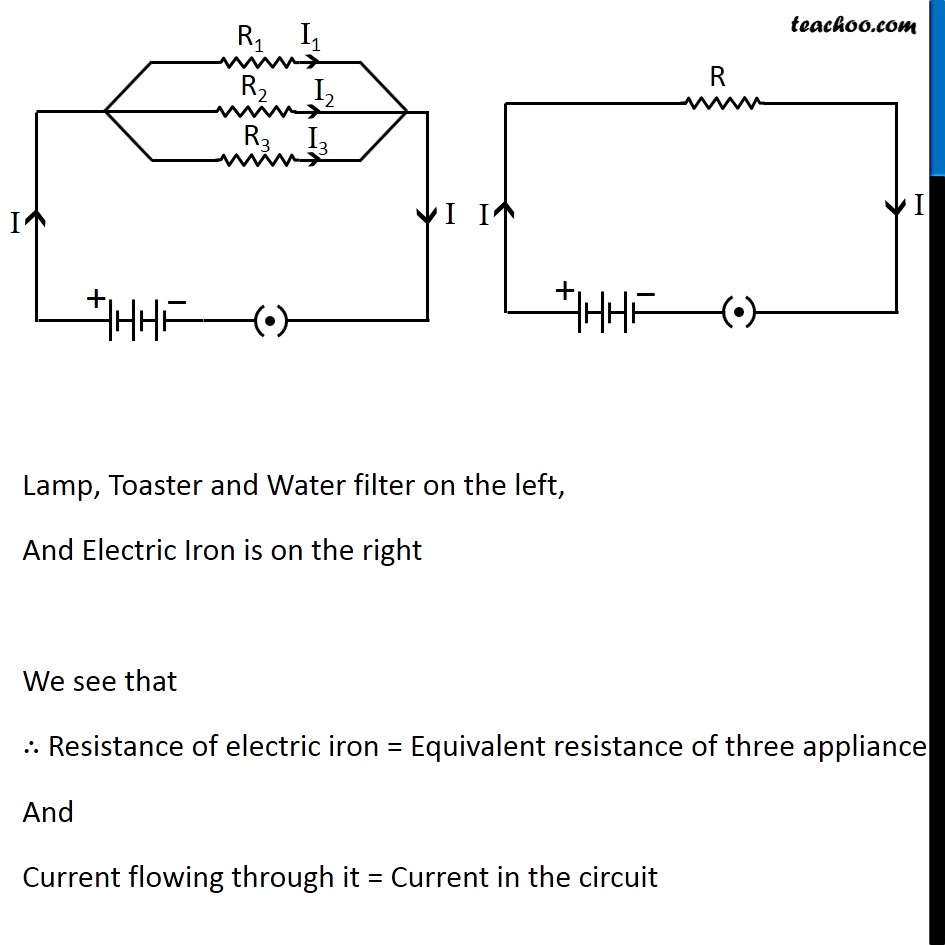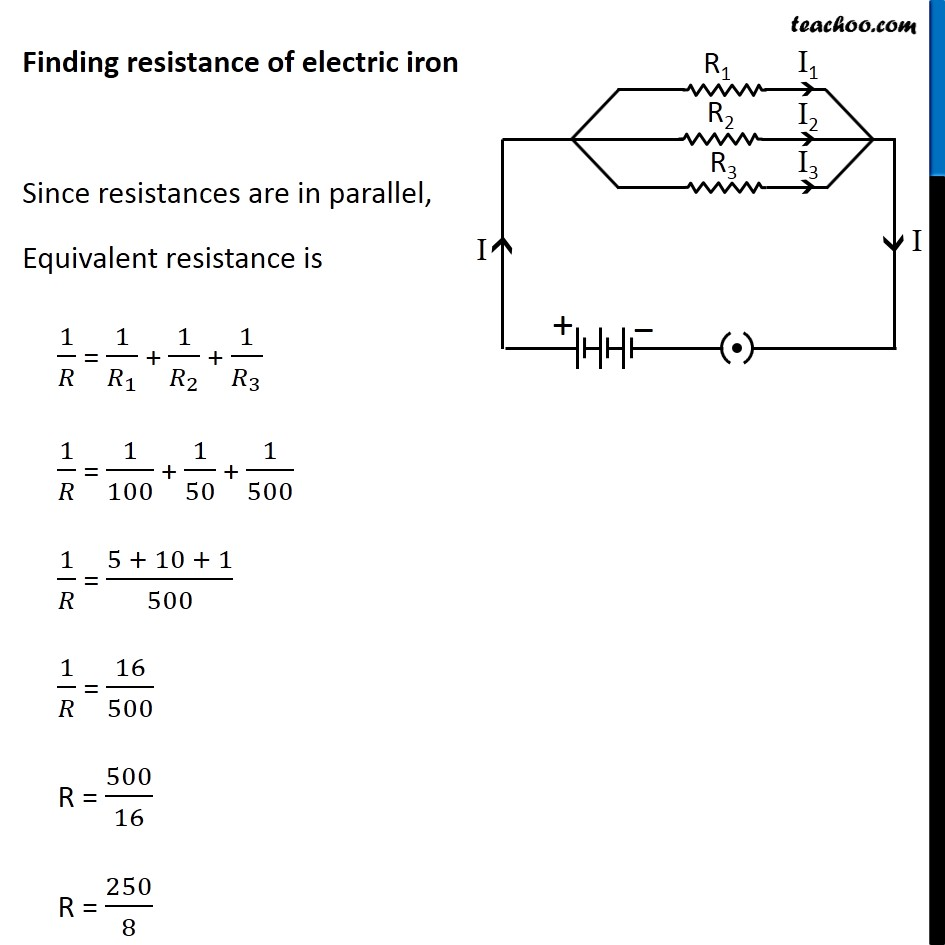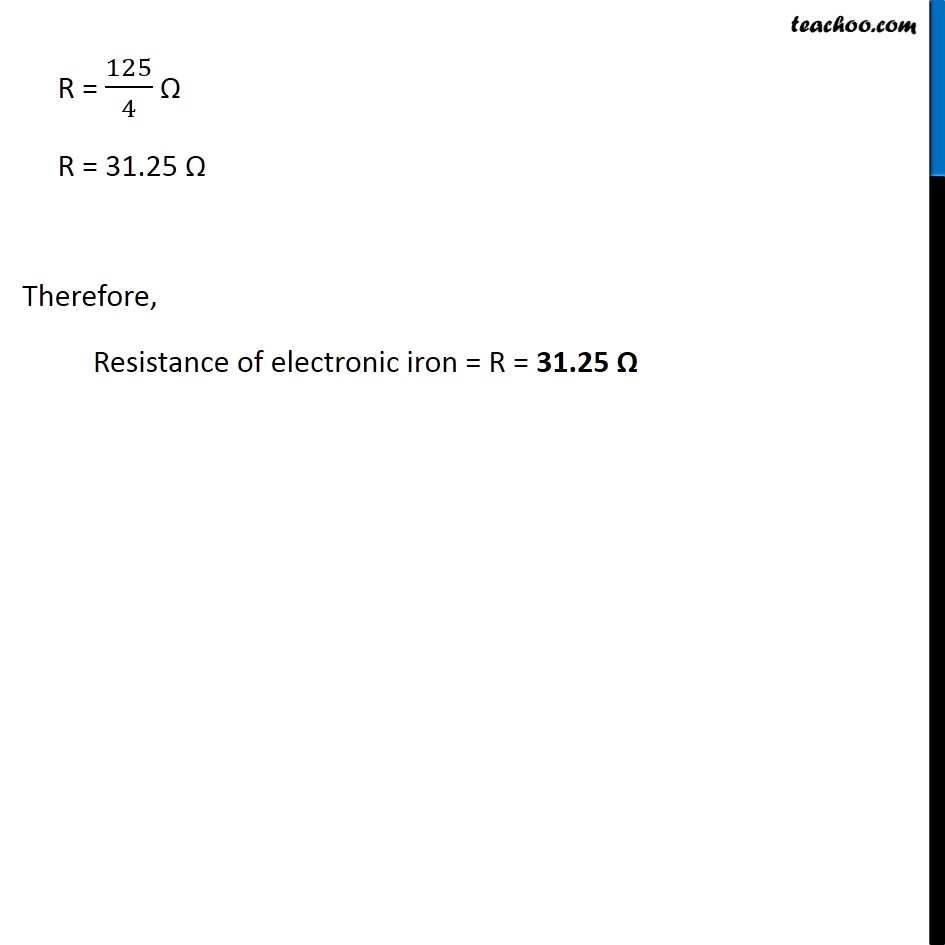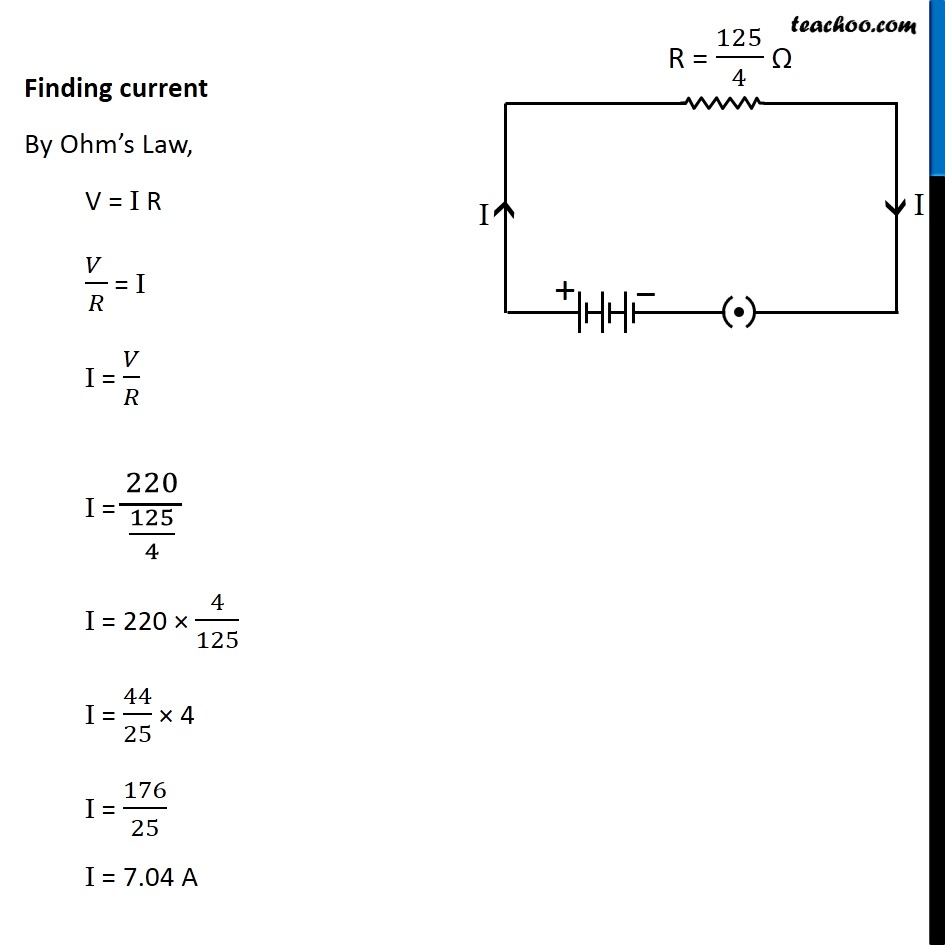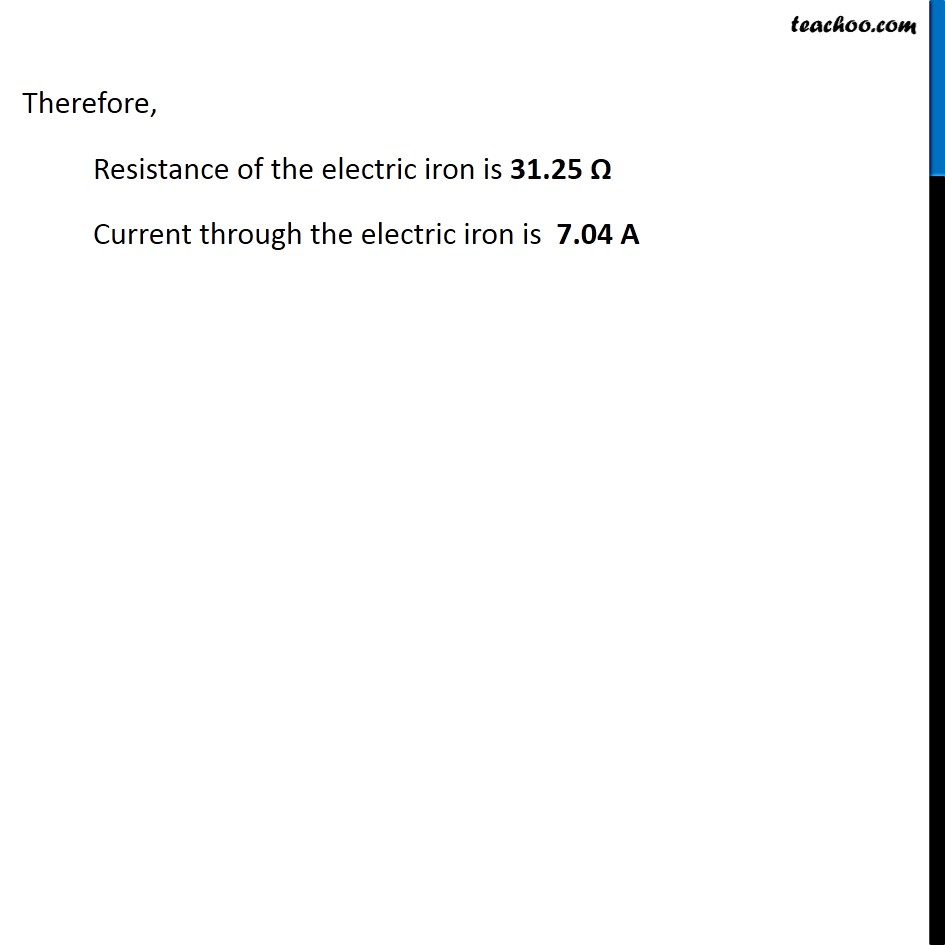Learn in your speed, with individual attention - Teachoo Maths 1-on-1 Class

### Transcript

Question 2 Page 216 An electric lamp of 100 Ω, a toaster of resistance 50 Ω, and a water filter of resistance 500 Ω are connected in parallel to a 220 V source. What is the resistance of an electric iron connected to the same source that takes as much current as all three appliances, and what is the current through it? Resistance of electric lamp = R1 = 100 Ω Resistance of toaster = R2 = 50 Ω Resistance of water filter = R3 = 500 Ω Potential difference = V = 220 V Lamp, Toaster and Water filter on the left, And Electric Iron is on the right We see that ∴ Resistance of electric iron = Equivalent resistance of three appliance And Current flowing through it = Current in the circuit Finding resistance of electric iron Since resistances are in parallel, Equivalent resistance is 1/𝑅 = 1/𝑅_1 + 1/𝑅_2 + 1/𝑅_3 1/𝑅 = 1/100 + 1/50 + 1/500 1/𝑅 = (5 + 10 + 1)/500 1/𝑅 = 16/500 R = 500/16 R = 250/8 R = 125/4 Ω R = 31.25 Ω Therefore, Resistance of electronic iron = R = 31.25 Ω Finding current By Ohm’s Law, V = I R (𝑉 )/𝑅 = I I = 𝑉/𝑅 I = 220/(125/4) I = 220 × 4/125 I = 44/25 × 4 I = 176/25 I = 7.04 A Therefore, Resistance of the electric iron is 31.25 Ω Current through the electric iron is 7.04 A